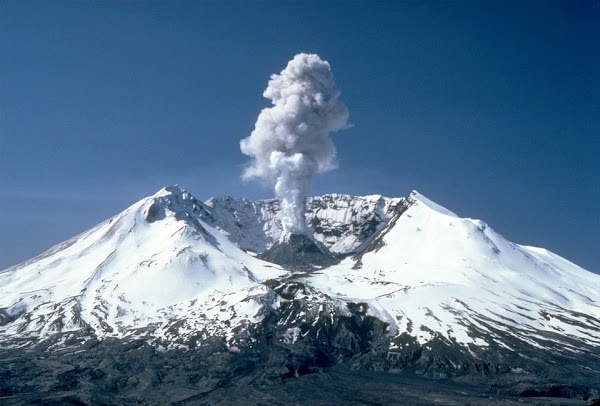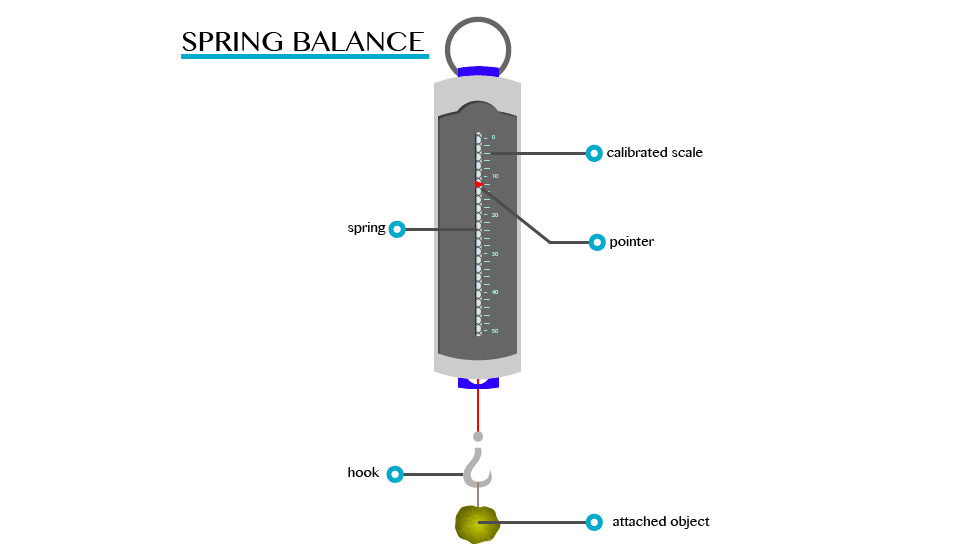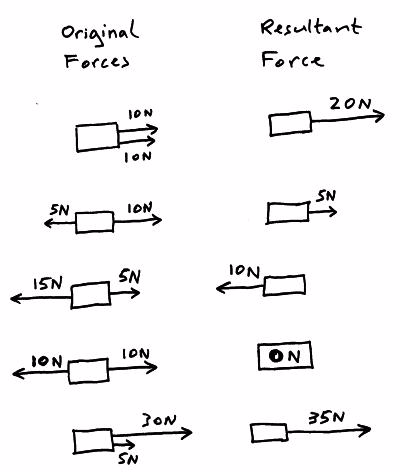## PHYSICS FORM ONE TOPIC 4: FORCE

### PHYSICS FORM ONE TOPIC 4: FORCEThursday, January 12, 2023
FORCE, A force is an interaction that causes a change. In mechanics, a force is an interaction that causes a change in velocity (an interaction that causes acceleration).

### The Concept of Force

Explain the concept of force

For better understanding of this concept, let’s ask ourselves the following question:
What causes/makes a body at rest to move?
What causes the same body in motion to stop?

The answers to these questions, is of course that a force is required to do so. Here, an applied force to a body can cause the body at rest to move or if already moving a body application of force can do the following when applied to a body.

Force can cause a change in the way the object moves
Change its size or shape
Change the direction in which an object is moving

A force is defined as a push or pulls experienced by an object. The force is usually described in terms of its size (magnitude) and direction.

The S.I Unit of Force

The SI unit of force is a Newton (N).

A Newton (1N) can be defined as the amount of force required to give a mass of one kilogram (1kg) an acceleration of 1m/s2

Measuring Instrument (Device)

The instrument that is used to measure the amount of force that is exerted on an object is called spring balance.

A spring balance can be used to measure small forces. It consists of a coiled spring fixed to the other end with a hook at the other end. The body upon which the force acts is attached to the hook. The distance through which the spring is stretched is directly proportional to the force applied by the balance.

A pointer is attached to the spring and the force is indicated on a calibrated scale.## Types of Force

#### Fundamental Types of Forces

There are two main types of forces namely:

1) Fundamental forces

2) Non fundamental forces

Fundamental Forces
Fundamental forces are the basic forces in nature that cannot be explained by the action of another force.

There are four types of fundamental forces. These include:
-The force of gravity
-The electromagnetic force
-The strong nuclear force
-The weak nucleus force

Force of gravity (weight)

The force of gravity is the pull by which the earth, moon and other very large bodies attract other objects towards themselves. It is commonly referred to as the weight of the object that is attracted.

All objects on the earth experience a force of gravity that is directed downwards towards centre of the earth. The earth’s gravitational pull is so great that it is able to hold most things firmly on the ground.

In mechanics, a freely falling body in the air moves down irrespective of its mass. This is due to force of gravity.

Conclusion, we can say that the force of gravity has the following properties.

It pulls (attracts) objects towards the centre of the earth.

It is directly proportional to the mass of the object. This means that the greater the mass the greater the pull of gravity.

It is strong when the mass is closer to the centre of the earth.

NB: It should be noted that the weight of an object is directly related to its Mass.

Weight, W = mass x g = mg

The g above has two meanings:
-It is the gravitation’s field strength (10N/Kg)
-It is the acceleration of free fall (10m/s2

Properties of gravitational forces

The following are the properties of gravitational force

-It always attracts objects.
-It is the weakest force among the four basic forces.
-It is a central force (gravitational force between two objects acts along the line joining the centres of the objects.
-It operates over very long distance.

Properties of electromagnetic force include

-It may be attractive or repulsive is nature
-It is a central force
-It is stronger than gravitational force
-It is also a long-range force (operates over a very long distance)

Properties of a strong nuclear force:

-It is basically an attractive force
-It is a non-central force (does not act at the centre)
-It is stronger than gravitational force
-It is a short-range force that is it operates only up to distance of the order of 10-14m

Properties of the weak nuclear force

-It is much stronger than the gravitational force but weaker than the strong nuclear force and electromagnetic force
-It acts on small ranges of up to 10-17m

Electromagnetic forceThis force includes both electric and magnetic force. It is relatively strong.

Strong nuclear force

This is the force, which holds of the constitutions (sub atomic particles) of the atomic nucleus. It acts within the nucleus of the atom.

Weak nuclear force

This is the force which appears only in certain nuclear processes.

### Effects of Force

The Effects of Forces

A force acting on an object may cause the object to change shape, to start moving, to stop moving, to accelerate or decelerate.

When two objects interact with each other they exert a force on each other, the forces are equal in size but opposite in direction.

Resultant force

The forces acting on an object can be replaced with a single force that causes the object to behave in the same way as all the separate forces acting together did, this one overall force is called theresultant force.If the resultant force acting on an object is ZERO then:
The object will remain stationary if it was stationary when the resultant force became zero.
Move at a constant (steady) speed in a straight line if it was moving when the resultant force became zero.

If the resultant force acting on an object is NOT ZERO then:
The object will accelerate or decelerate (speed up or slow down).

### The Effects of Forces on Materials

Example 3
A rectangular bar having a cross-sectional area of 80 mm2has a tensile force of 20 kN appliedto it. Determine the stress in the bar.Example 4
A circular section cable has a tensile force of 1 kN applied to it and the force produces a stress of7.8 MPa in the cable. Calculate the diameter of the cable.Example 5
A split pin requires a force of 400 N to shear it. The maximum shear stress before shear occurs is120 MPa. Determine the minimum diameter of the pin.PHYSICS FORM ONE TOPIC 4: FORCE
4/ 5
Oleh